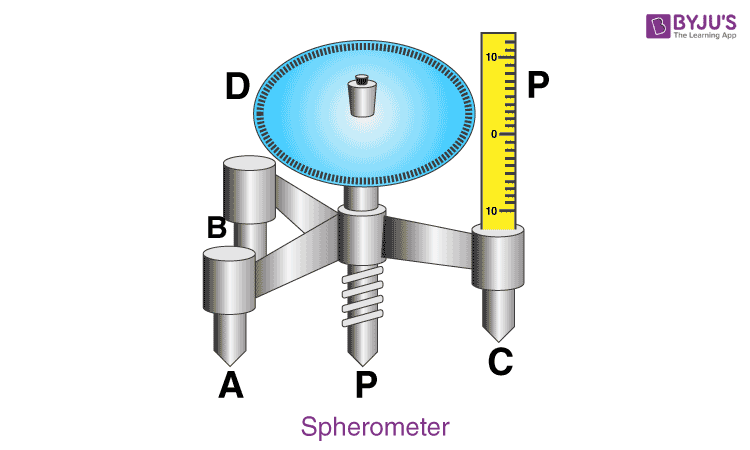# To Determine Radius Of Curvature Of A Given Spherical Surface By A Spherometer

A spherometer is a measuring device which has a metallic triangular frame supported on three legs. The tips of the three legs form an equilateral triangle and lie on the radius. There is a central leg which can be moved in a perpendicular direction. Below is the experiment on how to determine the radius of curvature of a given spherical surface by a spherometer.

## Aim

To determine the radius of curvature of a given spherical surface by a spherometer.

## Materials Required

1. Spherometer
2. Convex surface
3. A big size plane mirror

## Theory

### What is the least count of a spherometer?

Least count of the spherometer =

$$\begin{array}{l}\frac{pitch\;of\;the\;spherometer\;screw}{no.\;of\;divisions\;on\;the\;circular\;scale}\end{array}$$

The least count of the spherometer is 0.01 mm.

### What is the pitch of spherometer?

Pitch is defined as the distance moved by the central screw in one complete rotation of the circular disc scale. The pitch of the screw is 1 mm.

## Procedure

1. To get the three pricks of the spherometer, press the spherometer gently on the practical note-book by lifting the central screw and mark these pricks as A, B, and C.
2. Join the points A, B, and C to form a triangle and measure the distance between these points.
3. Note the distances between AB, BC, and CA and take their mean.
4. Find the value of the pitch which is the vertical scale division.
5. Record the pitch and least count of the spherometer.
6. Lift the screw sufficiently upwards.
7. Place the spherometer on the convex surface so that all the three legs rest on it.
8. Turn the screw gently downwards till it touches the convex surface.
9. Let a be the reading taken from the circular scale which is in line with the vertical scale.
10. Now remove and place the spherometer on the plane mirror.
11. Let n1 be the no.of rotations completed by the disc when it is moved downwards.
12. Continue rotating the disc till the tip of the screw touches the plane surface of the mirror.
13. Let b be the reading of the circular scale which is in line with the vertical scale.
14. From the last incomplete rotation, find the number of a circular scale division.
15. Repeat steps 6 to 14 three times and record the observations.

## Diagram## Observations

1. Distance between two legs of the spherometer
2. In △ABC marked by the legs of the spherometer

AB = …….cm

BC = …….cm

AC = …….cm

Mean value of

$$\begin{array}{l}\frac{AB+BC+CA}{3}=…..\;cm\end{array}$$
3. Least count of spherometer
4. 1 pitch scale division = 1 mm

No.of full rotations given to screw = 5

Distance moved by the screw = 5 mm

Hence, pitch, p = 5mm/5 = 1 mm

No.of divisions on circular (disc) scale = 100

Hence, least count = 1mm/100 = 0.01 mm = 0.001 cm

5. Table for sagitta (h)
 Sl.no Circular (disc) scale reading No.of complete rotations on a plane mirror (n1) No.of disc scale divisions in incomplete rotation x=(a-b) Total reading h=n1×p+×(L.C) (mm) 1. h1= 2. h2= 3. h3=

## Calculations

1. Find the value of h for every observation.
2. Find the mean value of h
3. Mean value of h =

$$\begin{array}{l}\frac{h_{1}+h_{2}+h_{3}}{3}=…..\;mm\end{array}$$
= ….. mm = ….cm

4. Calculate
5. $$\begin{array}{l}R=\frac{l^{2}}{6h}+\frac{h}{2}\;cm\end{array}$$

## Result

The radius of curvature of the given convex surface is ………..cm.

## Precautions

1. The movement of the screw should happen without any friction.
2. To avoid backlash error, the screw should be moved in the same direction.
3. Avoid excess rotation.

## Source Of Error

1. There might be friction in the screw.
2. There might be a back-lash error in the spherometer.
3. The divisions between the circular scale may not be of equal size.

## Viva Questions

Q1. What is the formula used to find the radius of curvature using spherometer?

Ans: Following is the formula used to find the radius of curvature using spherometer:

 $$\begin{array}{l}R=\frac{l^{2}}{6h}+\frac{h}{2}\end{array}$$

Q2. What is Len’s maker formula?

Ans: Following is the Len’s maker formula:

 $$\begin{array}{l}\frac{1}{f}=[\frac{n_{2}}{n_{1}}-1][\frac{1}{R_{1}}-\frac{1}{R_{2}}]\end{array}$$

Q3. What is the principle of spherometer?

Ans: The spherometer works on the principle of a micrometre screw.

Q4. Why is spherometer known by this name?

Ans: Spherometer is used to measure the radius of curvature of spherical surfaces. So it is known by that name.

Q5. What does the pitch of spherometer mean?

Ans: the pitch of spherometer is the distance between two consecutive threads of the screw which is parallel to the axis of rotation.